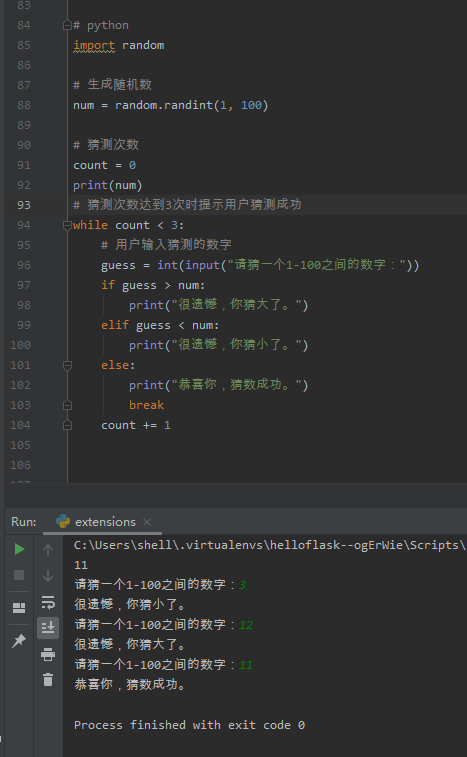•闪存
•博客
• 发言小组
• 投递新闻
• 提问博问
• 添加收藏
•文库

# python猜数游戏。求大佬帮忙解答一下，还没学到

0
[待解决问题]0

@鱼鱼96:@李珍拆: 好的，懂了

@鱼鱼96: 但这个写法是有很多问题的，只做理解，用于项目还需改进

0

python
import random

# 生成随机数

num = random.randint(1, 100)

# 用户输入猜测的数字

guess = int(input("请猜一个1-100之间的数字："))

count = 0

# 猜测次数达到3次时提示用户猜测成功

while count < 3:
if guess > num:
print("很遗憾，你猜大了。")
elif guess < num:
print("很遗憾，你猜小了。")
else:
print("恭喜你，猜数成功。")
break
count += 1

0

import random

# 生成一个1-100的随机数

number = random.randint(1, 100)

chances = 3

# 循环3次，提示用户猜数字

for i in range(chances):
# 用户输入一个数字
guess = int(input("请输入一个1-100之间的整数："))

``````# 判断猜测的数字与随机生成的数字的大小关系
if guess > number:
print("很遗憾，你猜大了")
elif guess < number:
print("很遗憾，你猜小了")
else:
print("恭喜你，猜数成功")
break
``````

# 如果3次机会用完仍未猜中，提示正确答案

if i == chances - 1 and guess != number:
print(f"很遗憾，你没有在{chances}次机会内猜中，正确答案是{number}")

0

python

import random

# 生成随机数

target_num = random.randint(1, 100)

# 设置可猜次数

guess_count = 3

print("猜数字游戏开始！")
while guess_count > 0:
guess = int(input("请输入一个1到100之间的整数："))
if guess < target_num:
print("很遗憾，你猜小了。")
elif guess > target_num:
print("很遗憾，你猜大了。")
else:
print("恭喜你，猜数成功！")
break
guess_count -= 1
print(f"你还有{guess_count}次机会。")

if guess_count == 0:
print(f"游戏结束，正确的数字是{target_num}。")

import random 引入 Python 的随机数模块。
target_num = random.randint(1, 100) 生成一个 1 到 100 之间的随机数。
guess_count = 3 设置可猜次数为 3。
while guess_count > 0: 开始循环，只要还有猜数机会就继续循环。
guess = int(input("请输入一个1到100之间的整数：")) 获取用户输入的猜测数字。
if guess < target_num: 判断用户猜测的数字是否小于随机数，如果是，输出猜小了的提示。
elif guess > target_num: 判断用户猜测的数字是否大于随机数，如果是，输出猜大了的提示。
else: 如果用户猜测的数字与随机数相等，输出猜数成功的提示并跳出循环。
guess_count -= 1 在每次循环结束时，将可猜次数减 1。
print(f"你还有{guess_count}次机会。") 输出剩余可猜次数的提示。
if guess_count == 0: 如果可猜次数用尽，输出游戏结束的提示并显示正确的数字。

Technologyforgood | 园豆：3643 (老鸟四级) | 2023-04-13 21:34

您需要登录以后才能回答，未注册用户请先注册